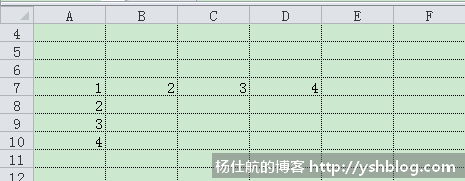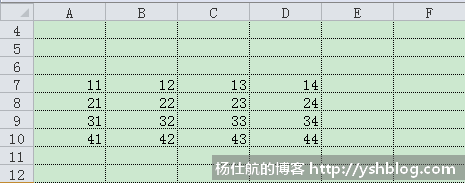### Python操作Excel

• 发布时间：2017年4月17日 11:21
• 作者：杨仕航
• 分类标签： Python
• 阅读(11497)
• 评论(0)

Python操作Excel，读写xls/xlsx文件已经有不少优秀的库。

win32com组件可以在https://sourceforge.net/projects/pywin32/files/pywin32下载，根据系统和Python版本选择对应的版本。下载安装完成之后，继续往下看内容。

#### 1、打开Excel文件

```#coding:utf-8
from win32com.client import Dispatch
import os

def open_xlsx(xlsx_path):
#把相对路径转成绝对路径
xlsx_fullname = os.path.abspath(xlsx_path)

#创建Excel对象
excel = Dispatch('excel.application')

#打开Excel文件
workbook = excel.workbooks.Open(xlsx_fullname)```

```#coding:utf-8
from win32com.client import DispatchEx
import os

def open_xlsx(xlsx_path):
#把相对路径转成绝对路径
xlsx_fullname = os.path.abspath(xlsx_path)

#创建Excel对象
excel = DispatchEx('excel.application')

#打开Excel文件
workbook = excel.workbooks.Open(xlsx_fullname)```

DispatchEx类可以创建新的Excel进程，不与其他进程造成干扰。

```#coding:utf-8
from win32com.client import DispatchEx
import os

def open_xlsx(xlsx_path):
#把相对路径转成绝对路径
xlsx_fullname = os.path.abspath(xlsx_path)

#创建Excel对象
pythoncom.CoInitialize()
excel = DispatchEx('excel.application')

#打开Excel文件
workbook = excel.workbooks.Open(xlsx_fullname)

pythoncom.CoUninitialize()```

pythoncom那两句是保护DispatchEx创建的进程。

```from win32com.client import Dispatch

excel = Dispatch('excel.application')
excel.visible = 1 #显示Excel```

```#coding:utf-8
from win32com.client import DispatchEx
import os

def open_xlsx(xlsx_path):
#把相对路径转成绝对路径
xlsx_fullname = os.path.abspath(xlsx_path)

#创建Excel对象
pythoncom.CoInitialize()
excel = DispatchEx('excel.application')
excel.visible = 0        #不显示Excel
excel.ScreenUpdating = 0 #关闭屏幕刷新

#打开Excel文件
workbook = excel.workbooks.Open(xlsx_fullname)

pythoncom.CoUninitialize()```

#### 2、关闭Excel文件，退出Excel进程

workbook对象有Close方法，第1个参数默认为False，关闭不保存。该方法使用可以参考Excel vba。

Excel退出进程，使用Application对象的Quit方法即可。

```#coding:utf-8
from win32com.client import DispatchEx
import os

def open_xlsx(xlsx_path):
#把相对路径转成绝对路径
xlsx_fullname = os.path.abspath(xlsx_path)

#创建Excel对象
pythoncom.CoInitialize()
excel = DispatchEx('excel.application')
excel.visible = 0        #不显示Excel
excel.ScreenUpdating = 0 #关闭屏幕刷新

#打开Excel文件
workbook = excel.workbooks.Open(xlsx_fullname)

#其他操作代码
#...

#关闭Excel文件，不保存(若保存，使用True即可)
workbook.Close(False)

#退出Excel
excel.Quit()
pythoncom.CoUninitialize()```

```#save_xlsx_path是保存的路径
workbook.SaveAs(os.path.abspath(save_xlsx_path))

#遍历退出
if excel:
for wrk in excel.workbooks:
wrk.Close(False)
excel.Quit()
pythoncom.CoUninitialize()```

#### 3、读写数据

```#假如已经打开了一个workbook
#workbook = ...

#激活第1个工作表
worksheet = workbook.worksheets
worksheet.Activate()

#获取当前工作表总行数
row_max = excel.Range("A56636").End(-4162).Row

#直接A列获取数据(该方法可以获取得到数据列表)
values = excel.Range("A1:A%s" % row_max).Value

#也可以遍历
for row in range(1, row_max+1):
print(worksheet.Cells(row, 1).Value)```

```from compiler.ast import flatten
flatten(worksheet.Range('A1:A5').value)```

```worksheet.Range('A7:A10').Value = [[1,],[2,],[3,],[4,]]
worksheet.Range('A7:D7').Value = [1,2,3,4]``````worksheet.Range('A7:D10').Value = [
[11, 12, 13, 14],
[21, 22, 23, 24],
[31, 32, 33, 34],
[41, 42, 43, 44]
]````worksheet.Range('A7').Value = '测试'`